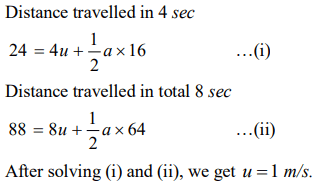## Motion in a Straight Line Questions and Answers Part-5

1. The acceleration of a moving body can be found from
a) Area under velocity-time graph
b) Area under distance-time graph
c) Slope of the velocity-time graph
d) Slope of distance-time graph

Explanation: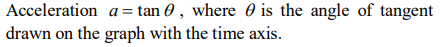2. The initial velocity of a particle is u (at t = 0) and the acceleration f is given by at . Which of the following relation is valid
a) $v=u+at^{2}$
b) $v=u+a\frac{t^{2}}{2}$
c) $v=u+at$
d) $v=u$

Explanation: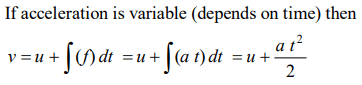3. The initial velocity of the particle is 10 m / sec and its retardation is $2m\diagup sec^{2}$ . The distance moved by the particle in 5th second of its motion is
a) 1 m
b) 19 m
c) 50 m
d) 75 m

Explanation: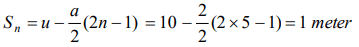4. A motor car moving with a uniform speed of 20 m / sec comes to stop on the application of brakes after travelling a distance of 10 m. Its acceleration is
a) $20m\diagup sec^{2}$
b) $-20m\diagup sec^{2}$
c) $-40m\diagup sec^{2}$
d) $+2m\diagup sec^{2}$

Explanation:5. The velocity of a body moving with a uniform acceleration of $2m\diagup sec^{2}$  is 10 m / sec . Its velocity after an interval of 4 sec is
a) $12 m\diagup sec$
b) $14 m\diagup sec$
c) $16 m\diagup sec$
d) $18 m\diagup sec$

Explanation: v = u + at = 10 + 2 * 4 = 18 m /sec

6. A particle starting from rest travels a distance x in first 2 seconds and a distance y in next two seconds, then
a) $Y=X$
b) $Y=2X$
c) $Y=3X$
d) $Y=4X$

Explanation: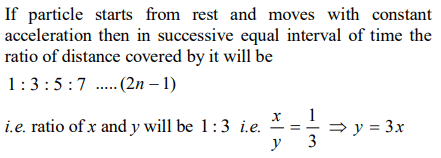7. The initial velocity of a body moving along a straight line is $7m\diagup s$ . It has a uniform acceleration of $4m\diagup s^{2}$. The distance covered by the body in the 5th second of its motion is
a) 25m
b) 35m
c) 50m
d) 85m

Explanation:8. The velocity of a body depends on time according to the equation $V=20+0.1t^{2}$   . The body is undergoing
a) Uniform acceleration
b) Uniform retardation
c) Non-uniform acceleration
d) Zero acceleration

Explanation: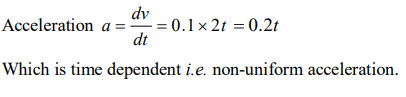9. Which of the following four statements is false
a) A body can have zero velocity and still be accelerated
b) A body can have a constant velocity and still have a varying speed
c) A body can have a constant speed and still have a varying velocity
d) The direction of the velocity of a body can change when its acceleration is constant